Courses

# Test: CPU & I/O Scheduling- 2

## 20 Questions MCQ Test Operating System | Test: CPU & I/O Scheduling- 2

Description
This mock test of Test: CPU & I/O Scheduling- 2 for Computer Science Engineering (CSE) helps you for every Computer Science Engineering (CSE) entrance exam. This contains 20 Multiple Choice Questions for Computer Science Engineering (CSE) Test: CPU & I/O Scheduling- 2 (mcq) to study with solutions a complete question bank. The solved questions answers in this Test: CPU & I/O Scheduling- 2 quiz give you a good mix of easy questions and tough questions. Computer Science Engineering (CSE) students definitely take this Test: CPU & I/O Scheduling- 2 exercise for a better result in the exam. You can find other Test: CPU & I/O Scheduling- 2 extra questions, long questions & short questions for Computer Science Engineering (CSE) on EduRev as well by searching above.
QUESTION: 1

### For the processes listed in the following table, which of the following scheduling schemes will give the lowest average turnaround time? Process    Arrival Time    Processing Time   A                  0                      3   B                 1                       6   C                 4                       4   D                 6                       2

Solution:

Turnaround time is the total time taken between the submission of a program/process/thread/task (Linux) for execution and the return of the complete output to the customer/user. Turnaround Time = Completion Time - Arrival Time. FCFS = First Come First Serve (A, B, C, D) SJF = Non-preemptive Shortest Job First (A, B, D, C) SRT = Shortest Remaining Time (A(3), B(1), C(4), D(2), B(5)) RR = Round Robin with Quantum value 2 (A(2), B(2), A(1),C(2),B(2),D(2),C(2),B(2)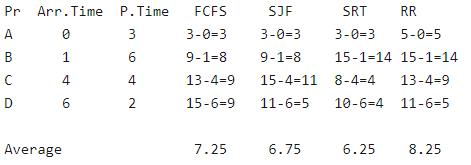QUESTION: 2

### Which of the following is FALSE about SJF (Shortest Job First Scheduling)? S1: It causes minimum average waiting time S2: It can cause starvation

Solution:
1. Both SJF and Shortest Remaining time first algorithms may cause starvation. Consider a situation when long process is there in ready queue and shorter processes keep coming.
2. SJF is optimal in terms of average waiting time for a given set of processes, but problems with SJF is how to know/predict time of next job.
QUESTION: 3

### Consider an arbitrary set of CPU-bound processes with unequal CPU burst lengths submitted at the same time to a computer system. Which one of the following process scheduling algorithms would minimize the average waiting time in the ready queue?

Solution:

Turnaround time is the total time taken by the process between starting and the completion and waiting time is the time for which process is ready to run but not executed by CPU scheduler. As we know, in all CPU Scheduling algorithms, shortest job first is optimal i.ie. it gives minimum turn round time, minimum average waiting time and high throughput and the most important thing is that shortest remaining time first is the pre-emptive version of shortest job first. shortest remaining time first scheduling algorithm may lead to starvation because If the short processes are added to the cpu scheduler continuously then the currently running process will never be able to execute as they will get pre-empted but here all the processes are arrived at same time so there will be no issue such as starvation. So, the answer is Shortest remaining time first, which is answer (A).

QUESTION: 4

Consider the following processes, with the arrival time and the length of the CPU burst given in milliseconds. The scheduling algorithm used is preemptive shortest remaining-time first.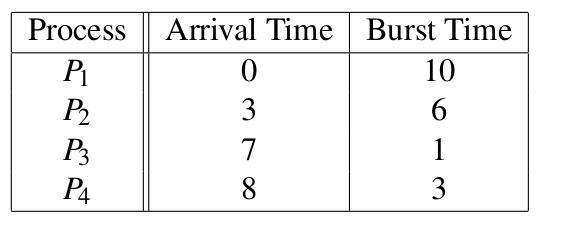The average turn around time of these processes is ___________ milliseconds.   Note : This question was asked as Numerical Answer Type.

Solution:

PreEmptive Shortest Remaining time first scheduling, i.e. that processes will be scheduled on the CPU which will be having least remaining burst time( required time at the CPU). The processes are scheduled and executed as given in the below Gantt chart.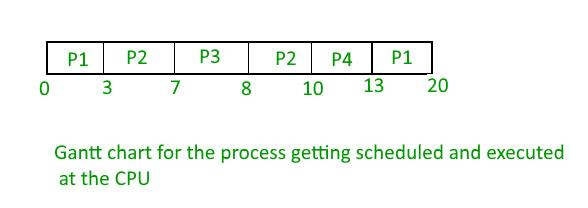Turn Around Time(TAT) = Completion Time(CT) - Arrival Time(AT) TAT for P1 = 20 - 0 = 20 TAT for P2 = 10 - 3 = 7 TAT for P3 = 8- 7 = 1 TAT for P4 = 13 - 8 = 5 Hence, Average TAT = Total TAT of all the processes / no of processes = ( 20 + 7 + 1 + 5 ) / 4 = 33 / 4 = 8.25   Thus, A is the correct choice.

QUESTION: 5

Assume every process requires 3 seconds of service time in a system with single processor. If new processes are arriving at the rate of 10 processes per minute, then estimate the fraction of time CPU is busy in system?

Solution:

10 processes -> 1 min
1 process-> 1/10 min = 6 sec (Arrival rate)
Each process -> 3 sec service time 3/6 * 100 = 50% of time CPU is busy.

QUESTION: 6

When an interrupt occurs, an operating system

Solution:

Scheduler decides that the interrupted process will complete execution or some other process will be executed. If the interrupt signaled an I/O completion event, and at the same time a high priority process came into Ready state then the scheduler block the interrupted process and dispatch the high priority process in the running state. If low priority process comes into Ready state then scheduler dispatch the interrupted process. Hence, D is correct.

QUESTION: 7

Consider the following CPU processes with arrival times (in milliseconds) and length of CPU bursts (in milliseconds) except for process P4 as given below:
Process    Arrival Time    Burst Time
P1                  0                  5
P2                  1                  1
P3                  3                  3
P4                  4                  x
If the average waiting time across all processes is 2 milliseconds and pre-emptive shortest remaining time first scheduling algorithm is used to schedule the processes, then find the value of x ?

Solution:

If we take value of x is 2, then we have gantt chart as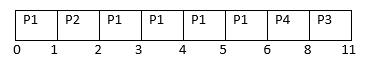So, completion time of P1, P2, P3, and P4 are 6, 2, 11, and 8 respectively. Turn around time of P1, P2, P3, and P4 are 6, 1, 8, and 4 respectively. Waiting time of P1, P2, P3, and P4 are 1, 0, 5, and 2 resectively. Therefore, average waiting time = (1+0+5+2) / 4 = 8/2 = 2 Option (B) is correct.

QUESTION: 8

Consider the following four processes with the arrival time and length of CPU burst given in milliseconds :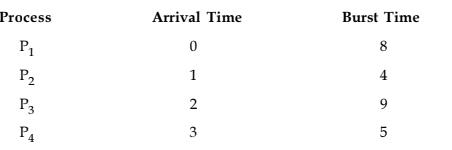The average waiting time for preemptive SJF scheduling algorithm is __________.

Solution:

First we will make gantt chart of given process then we will calculate turn around time and waiting time of individual process.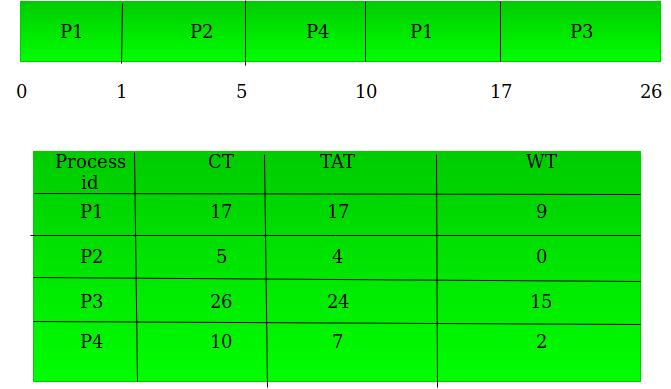Now we have to calculate average waiting time for schedule:avg waiting time = wt(P1 + P2 + P3 + P4 )/number of process.    ie.

(9 + 0 + 15 + 2) / 4
= 26 / 4
= 6.5

QUESTION: 9

Which module gives control of the CPU to the process selected by the short - term schedular ?

Solution:

There are three type of scheduler:

1. Short-term scheduler
2. Mid-term scheduler
3. Long-term scheduler

Dispatcher is responsible for handovering the control of CPU to the newly selected process by the Short-term scheduler. Refer: Process Scheduler So, option (A) is correct.

QUESTION: 10

Which of the following scheduling algorithms may cause starvation ? a. First-come-first-served b. Round Robin c. Priority d. Shortest process next e. Shortest remaining time first

Solution:
1. In First Come First Serve(FCFS) if a process with a very large Burst Time comes before other processes, the other process will have to wait for a long time but it is clear that other process will definitely get their chance to execute, so it will not suffer from starvation.
2. In Round Robin there is a fixed time quant and every process will get their chance to be executed, so no starvation is here.
3. In Priority based scheduling if higher priority process keep on coming then low priority process will suffer from starvation.
4. In Shortest Job First(SJF) if process with short process time keep on coming continuously then process with higher burst time will do wait and suffer from starvation.
5. In Shortest remaining time first(SRTF) process with shortest burst time will execute first because of this process with high burst time may suffer from starvation.

So, option (C) is correct.

QUESTION: 11

Which of the following statement is true?

Solution:

Jitter is the variation / displacement between the signals or data being sent. Hard real operating systems deal with more sensitive systems which require strict time deadlines like engine control systems, satellite launching systems etc while soft real operating systems do not require strict timing constraints and a bit delay is permissible, like mobile phones, online database systems. So hard real operating systems require minimised jitter.

QUESTION: 12

Consider three CPU intensive processes P1, P2, P3 which require 20, 10 and 30 units of time, arrive at times 1, 3 and 7 respectively. Suppose operating system is implementing Shortest Remaining Time first (preemptive scheduling) algorithm, then _____ context switches are required (suppose context switch at the beginning of Ready queue and at the end of Ready queue are not counted).

Solution: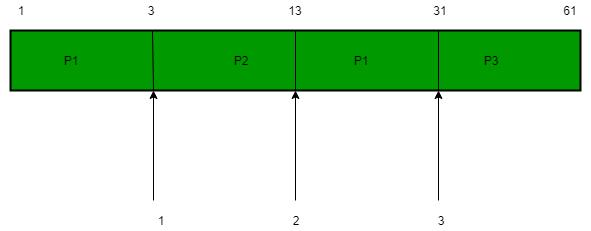There are 3 context switch. So. option (A) is correct.

QUESTION: 13

Five jobs A, B, C, D and E are waiting in Ready Queue. Their expected runtimes are 9, 6, 3, 5 and x respectively. All jobs entered in Ready queue at time zero. They must run in _____ order to minimize average response time if 3 < x < 5.

Solution:

We will solve it by minimizing the avg Waiting Time and Take x = 4 BT = Burst or Execution time CT = completion time P = Process AWT = Average Waiting Time AT = Arrival time WT = Waiting time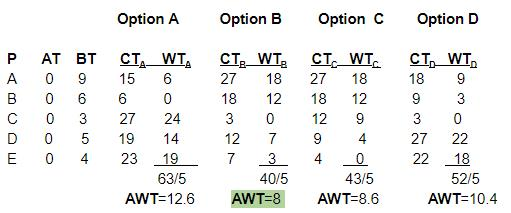We get minimum average waiting time from the sequence given in option (B)

QUESTION: 14

Which of the following is not an optimization criterion in the design of a CPU scheduling algorithm?

Solution:

Minimum CPU utilization is not an optimization criterion as various optimization techniques and scheduling algorithms are used to bring the best CPU performance.

QUESTION: 15

Below is the precedence graph for a set of tasks to be executed on a parallel processing system S.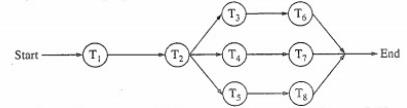What is the efficiency of this precedence graph on S if each of the tasks T1, T2, T3,....T8 takes the same time and the system S has five processors?

Solution:

It can be seen that along with the sequential execution of T1 and T2, (T3, T6), (T4, T7) and (T5, T8), all these three processes can be executed in parallel. So total number of processes that can be executed in 4 units time using 5 available processors = 5*4 = 20 But here processes that are executing in 4 units time = 8 Throughput = 8/20 * 100 = 40% So, option (B) is correct.

QUESTION: 16

A scheduling Algorithm assigns priority proportional to the waiting time of a process. Every process starts with priority zero (lowest priority). The scheduler reevaluates the process priority for every ‘T’ time units and decides next process to be scheduled. If the process have no I/O operations and all arrive at time zero, then the scheduler implements _________ criteria.

Solution:

A scheduling Algorithm assigns priority proportional to the waiting time of a process. Every process starts with priority zero (lowest priority). The scheduler reevaluates the process priority for every ‘T’ time units and decides next process to be scheduled. If the process have no I/O operations and all arrive at time zero, then the scheduler implements Round Robin Scheduling criteria. So, option (B) is correct.

QUESTION: 17

In a system using single processor, a new process arrives at the rate of six processes per minute and each such process requires seven seconds of service time. What is the CPU utilization?

Solution:

Number of processes per minute = 6 Burst time of each process = 7 secs
CPU utilization time within a minute = 6*7 = 42 secs
% CPU utilization = useful time / total time * 100
= (42/60) * 100
70%
Option (A) is correct.

QUESTION: 18

Consider the following justifications for commonly using the two-level CPU scheduling: I. It is used when memory is too small to hold all the ready processes. II. Because its performance is same as that of the FIFO. III. Because it facilitates putting some set of processes into memory and a choice is made from that. IV. Because it does not allow to adjust the set of in-core processes. Which of the following is true ?

Solution:

The two-level CPU scheduling is used when memory is too small to hold all the ready processes because it facilitates putting some set of processes into memory and a choice is made from that. So, option (D) is correct.

QUESTION: 19

Which of the following statements is not true for Multi Level Feedback Queue processor scheduling algorithm?

Solution:

For Multi Level Feedback Queue processor scheduling algorithm:

• Queues have different priorities
• Each queue may have different scheduling algorithm
• Processes are not permanently assigned to a queue.
• This algorithm can be configured to match a specific system under design
QUESTION: 20

In which of the following scheduling criteria, context switching will never take place ?

Solution:

In Non - preemptive algorithms context switching will never take place because it doesn't allow to switch the process until it is completed fully. So, option (C) is correct.